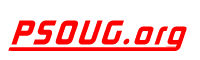Quick Search: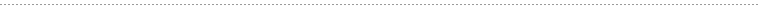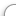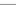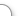CODE Oracle PL/SQL Code Library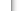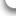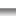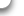JOBS Find Or Post Oracle JobsFORUM Oracle Discussion & ChatThe Oracle PL/SQL REGR_SLOPE Function      [Return To Index] Jump to: Select a Location PSOUG Home Page Oracle Code Library mySQL Code Library PHP Code Library JavaScript Code Library Oracle Terms & Definitions Oracle Error Codes PSOUG Community Blogs Oracle Jobs Board PSOUG Forum Oracle User Group Directory Free Oracle Magazines Online Learning Center PSOUG Presentations Advanced Code Search News and Events Sponsors Page Submit Code Contact UsLooking for the original pages? (formerly called "Morgan's Library") You can find them here.# Term: REGR_SLOPE

Definition:
In Oracle, REGR_SLOPE is a linear regression analytic function which is used to measure the slope of a line. Its computation formula looks like this:

COVAR_POP(x,y) / VAR_POP(y)

It accepts two numeric inputs. Note that the pairs having any argument's value as NULL are eliminated, but the final result might be NULL as per the above computation.

Example Syntax:

REGR_R2(x,y)

In the syntax, 'x' is referred to as the dependent variable and 'y' is referred to as the independent variable of the Regression Line.

Example Usage:

The SQL query below uses REGR_SLOPE to measure the slope of line formed by the columns DEPTNO and SAL.

```SELECT deptno, sal, REGR_SLOPE(deptno, sal) OVER (partition by deptno) "REGR_SLOPE"
FROM employee```